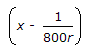# Civil Engineering - Structural Design Specifications

Exercise : Structural Design Specifications - Section 1
11.
The maximum area of compression reinforcement in a beam of cross section B x D is limited to
0.02 BD
0.03 BD
0.04 BD
0.05 BD
Explanation:
No answer description is available. Let's discuss.

12.
Moist curing of the exposed surfaces of concrete is done at least for
3 days
5 days
7 days
14 days
Explanation:
No answer description is available. Let's discuss.

13.
Pick up the incorrect statement from the following:
The gross section of the web of rolled I-beams is the depth of the beam multiplied by the web thickness
The gross section of the web of channels is the depth of the web multiplied by its thickness
The gross section of the web of plate girders is the depth of the web plate multiplied by its thinkness
None of these.
Explanation:
No answer description is available. Let's discuss.

14.
If the ratio of effective span to overall depth of a simply supported beam is equal or greater than 1 but less than or equal to 2, the lever arm, is
0.2 (l + 2D)
0.3 (l + 3D)
0.2 (l + 3D)
0.3 (l + 2D)
If the slenderness ratio of a column is more than 160, the compressive stress obtained by the secant formula is multiplied by a factorin which value of x is